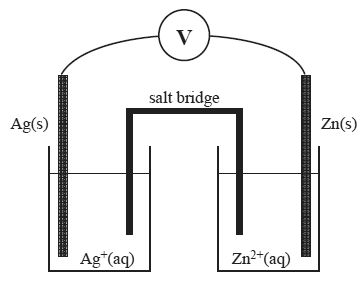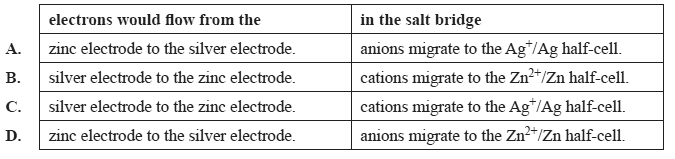Galvanic cells(2012 VCE) 1) A galvanic cell set up under standard conditions is shown below.Which one of the following is correct? As the cell dischargesSolutionb) In this cell A. Ag+(aq) is reduced and the Zn(s) is oxidised. B. Ag(s) is oxidised and the Zn2+(aq) is reduced. C. Ag(s) is reduced and the Zn2+(aq) is oxidised. D. Ag+(aq) is oxidised and the Zn(s) is reduced. Solutionc) The cathode in this cell and the maximum voltage produced by this cell, under standard conditions, are respectively A. Ag and 0.16 V B. Ag and 1.56 V C. Zn and 0.16 V D. Zn and 1.56 V Solution2) Which one of the following statements is true for both galvanic cells and electrolytic cells? A. Reduction occurs at the negative electrode in both cells. B. Reduction occurs at the cathode in both cells. C. Anions migrate to the cathode in both cells. D. The anode is positive in both cells. Solution